Visitors Online: 77 | Monday 23rd September 2019
 CBSE Guess > Papers > Question Papers > Class XII > 2005 > Maths > Compartment Delhi Set-II MATHEMATICS 2005 (Set II—Compartment Delhi)
Except for the following questions, all the remaining questions have been asked in maths I . SECTION - A Q. 1. Using properties of determinants, show thatQ. 7. Evaluate: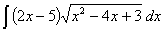Q. 13. Differentiate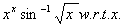Q. 15. Find the intervals in which the function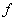given byis (a) strictly increasing, (b) strictly decreasing. Q. 16. Using integration, find the area of the smaller region bounded by the curve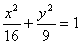and the straight lineSECTION - B Q. 19. Using vectors, prove that the median to the base of an isosceles triangle is perpendicular to the base. Q. 21. A man can throw a stone to a maximum distance of 125 m. for how much time will it remain in air and up to what height will it rise? (Take g = 9.8 m/s 2 ) Q. 24. Two unlike parallel forcesact at two points 'x' units apart. If the direction of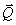is reversed them prove that the resultant is displayed through the distanceunits. SECTION - C Q. 19. An experiment succeeds twice as often as it fails. Find the probability that in the next six trials, there will be at leas 4 successes. Q. 23. In the beginning of each quarter Rs. 500 are deposited into a savings account that pays 8% per annum compounded quarterly. Find the balance in this account after 6 years. Maths 2005 Question Papers Class XII Delhi Outside Delhi Compartment Delhi Compartment Outside Delhi Set I Set I Set I Set I Set II Set II Set II Set II Set III Set III CBSE 2005 Question Papers Class XII